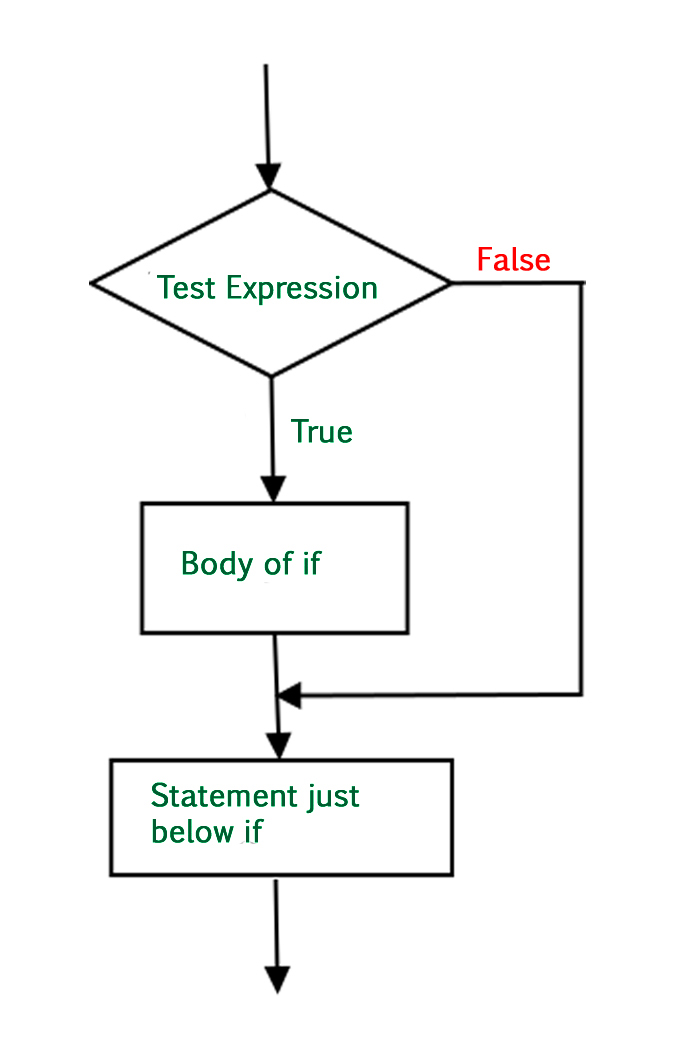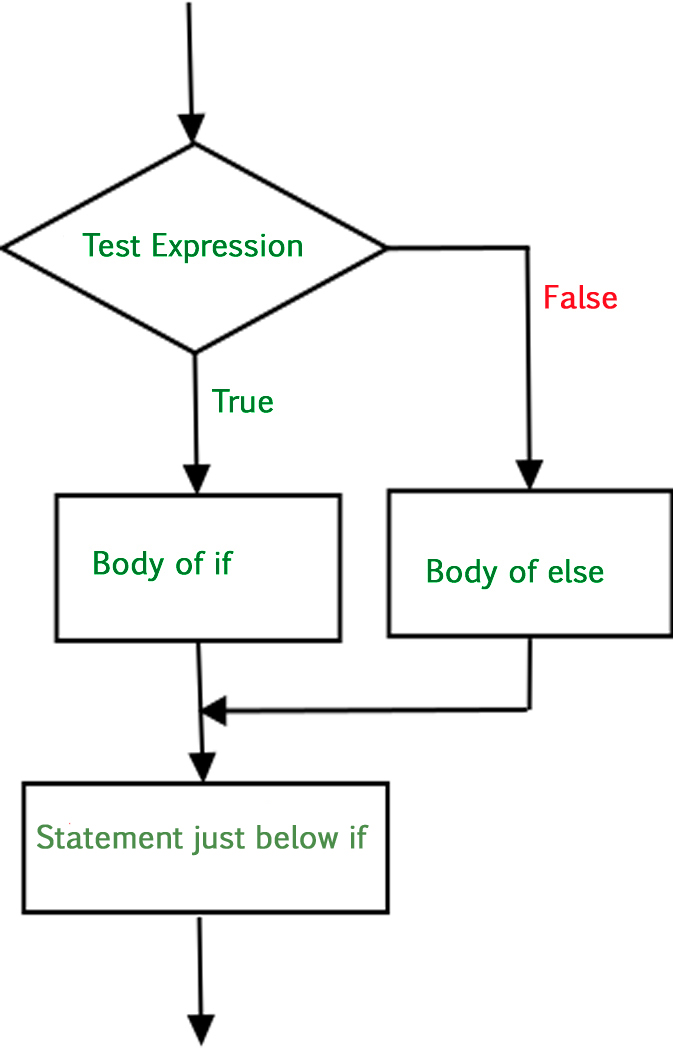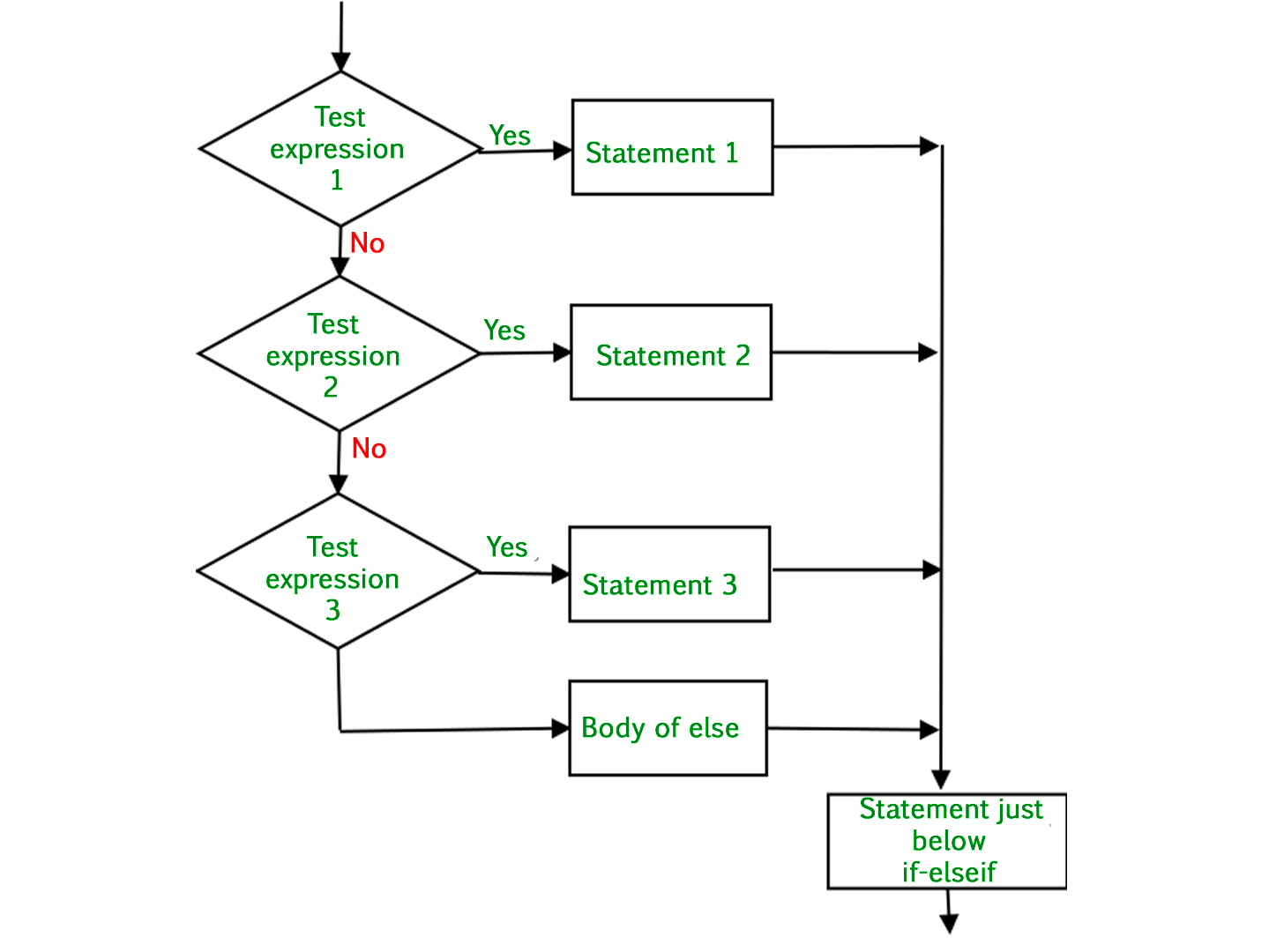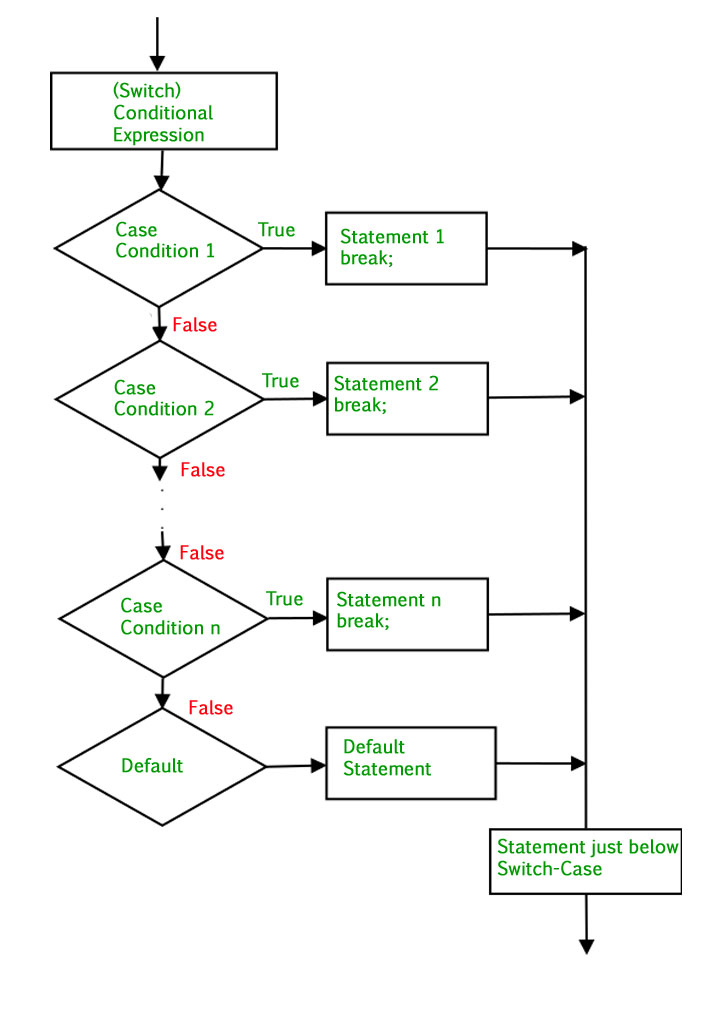# PHP | Decision Making

PHP allows us to perform actions based on some type of conditions that may be logical or comparative. Based on the result of these conditions i.e., either TRUE or FALSE, an action would be performed as asked by the user. It’s just like a two- way path. If you want something then go this way or else turn that way. To use this feature, PHP provides us with four conditional statements:

• if statement
• if…else statement
• if…elseif…else statement
• switch statement

Let us now look at each one of these in details:

1. if Statement: This statement allows us to set a condition. On being TRUE, the following block of code enclosed within the if clause will be executed.

Syntax :

```if (condition){
// if TRUE then execute this code
}
```

Example:

 ` 0) { ` `    ``echo` `"The number is positive"``; ` `} ` `?> `

Output:

```The number is positive
```

Flowchart:2. if…else Statement: We understood that if a condition will hold i.e., TRUE, then the block of code within if will be executed. But what if the condition is not TRUE and we want to perform an action? This is where else comes into play. If a condition is TRUE then if block gets executed, otherwise else block gets executed.

Syntax:

```if (condition) {
// if TRUE then execute this code
}
else{
// if FALSE then execute this code
}
```

Example:

 ` 0) { ` `    ``echo` `"The number is positive"``; ` `} ` ` `  `else``{ ` `    ``echo` `"The number is negative"``; ` `} ` `?> `

Output:

```The number is negative
```

Flowchart:3. if…elseif…else Statement: This allows us to use multiple if…else statements. We use this when there are multiple conditions of TRUE cases.
Syntax:

```if (condition) {
// if TRUE then execute this code
}
elseif {
// if TRUE then execute this code
}
elseif {
// if TRUE then execute this code
}
else {
// if FALSE then execute this code
}
```

Example:

 ` `

Output:

```Happy Independence Day!!!
```

Flowchart:4. switch Statement: The “switch” performs in various cases i.e., it has various cases to which it matches the condition and appropriately executes a particular case block. It first evaluates an expression and then compares with the values of each case. If a case matches then the same case is executed. To use switch, we need to get familiar with two different keywords namely, break and default.

1. The break statement is used to stop the automatic control flow into the next cases and exit from the switch case.
2. The default statement contains the code that would execute if none of the cases match.

Syntax:

```switch(n) {
case statement1:
code to be executed if n==statement1;
break;
case statement2:
code to be executed if n==statement2;
break;
case statement3:
code to be executed if n==statement3;
break;
case statement4:
code to be executed if n==statement4;
break;
......
default:
code to be executed if n != any case;

```

Example:

 ` `

Output:

```Its February
```

Flowchart:Ternary Operators

In addition to all this conditional statements, PHP provides a shorthand way of writing if…else, called Ternary Operators. The statement uses a question mark (?) and a colon (:) and takes three operands: a condition to check, a result for TRUE and a result for FALSE.
Syntax:

`(condition) ? if TRUE execute this : otherwise execute this;`

Example:

 ` 0) { ` `    ``echo` `"The number is positive \n"``; ` `} ` `else` `{ ` `    ``echo` `"The number is negative \n"``; ` `} ` ` `  `// This whole lot can be written in a  ` `// single line using ternary operator ` `echo` `(``\$x` `> 0) ? ``'The number is positive'` `:  ` `                ``'The number is negative'``; ` `?> `

Output:

```The number is negative
The number is negative
```

If you like GeeksforGeeks and would like to contribute, you can also write an article using write.geeksforgeeks.org or mail your article to review-team@geeksforgeeks.org. See your article appearing on the GeeksforGeeks main page and help other Geeks.